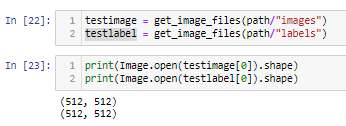# How to use unet_learner with only 1 channel?

Hi all, a continuation of my previous attempt (still a newbie now moving on to lesson4). Now trying to get the unet_learner to run with 1 channel input.
For reference, both my masks and my images are simple 1channel .tif files.Code I am running, arch= various
``````import fastbook
fastbook.setup_book()
from fastbook import *
path =Path("D:/pytorch/data/2D_Zebrafish/fastai")
codes = ['Zebrafish']

path, bs=12, fnames = get_image_files(path/"images"),
label_func = lambda o: path/'labels'/f'{o.stem}_annotationLabels.tif',
codes =codes, num_workers=0
)

def custom_resnet18(*args, **kwargs):
model = resnet18(*args, **kwargs)
model.conv1 = nn.Conv2d(1, 64, kernel_size=7, stride=2, padding=3, bias=False)
return model

#####ERROR WHY NIN=3####
learn = unet_learner(dls, arch = ???, n_in=1, n_out=1, loss_func=MSELossFlat(), lr=0.0001, normalize=False)
learn.fine_tune(1)
``````

I am getting an error similar to this post, but following the instructions there for a custom model does not change the result. I also am unable to load another pretrained unet using:

``````model = torch.hub.load('mateuszbuda/brain-segmentation-pytorch', 'unet', in_channels=1, out_channels=1, init_features=8, pretrained=False)
``````

I can get that model to work for my data using standard PyTorch. I would really like to learn to use FastAI, though.

Plugging resnet18 into the arch gives me:

``````RuntimeError: Given groups=1, weight of size [64, 1, 7, 7], expected input[12, 3, 512, 512] to have 1 channels, but got 3 channels instead
``````

While plugging in the custom_resnet18 defined above and in the linked post gives:

``````AssertionError: Unexpected number of input channels, found 1 while expecting 3
``````

That is the same as the original error in the above post, so that thread did not resolve the issue for me.

Full error
``````---------------------------------------------------------------------------
AssertionError                            Traceback (most recent call last)
Input In , in <cell line: 19>()
16     return model
18 #####ERROR WHY NIN=3####
---> 19 learn = unet_learner(dls, custom_resnet18, n_in=1, n_out=1, loss_func=MSELossFlat(), lr=0.0001, normalize=False)
20 learn.fine_tune(1)

File D:\Anaconda\envs\fastai2\lib\site-packages\fastai\vision\learner.py:245, in unet_learner(dls, arch, normalize, n_out, pretrained, config, loss_func, opt_func, lr, splitter, cbs, metrics, path, model_dir, wd, wd_bn_bias, train_bn, moms, **kwargs)
243 img_size = dls.one_batch().shape[-2:]
244 assert img_size, "image size could not be inferred from data"
--> 245 model = create_unet_model(arch, n_out, img_size, pretrained=pretrained, **kwargs)
247 splitter=ifnone(splitter, meta['split'])
248 learn = Learner(dls=dls, model=model, loss_func=loss_func, opt_func=opt_func, lr=lr, splitter=splitter, cbs=cbs,
249                metrics=metrics, path=path, model_dir=model_dir, wd=wd, wd_bn_bias=wd_bn_bias, train_bn=train_bn,
250                moms=moms)

File D:\Anaconda\envs\fastai2\lib\site-packages\fastai\vision\learner.py:220, in create_unet_model(arch, n_out, img_size, pretrained, cut, n_in, **kwargs)
218 "Create custom unet architecture"
219 meta = model_meta.get(arch, _default_meta)
--> 220 body = create_body(arch, n_in, pretrained, ifnone(cut, meta['cut']))
221 model = models.unet.DynamicUnet(body, n_out, img_size, **kwargs)
222 return model

File D:\Anaconda\envs\fastai2\lib\site-packages\fastai\vision\learner.py:77, in create_body(arch, n_in, pretrained, cut)
75 "Cut off the body of a typically pretrained `arch` as determined by `cut`"
76 model = arch(pretrained=pretrained)
---> 77 _update_first_layer(model, n_in, pretrained)
78 if cut is None:
79     ll = list(enumerate(model.children()))

File D:\Anaconda\envs\fastai2\lib\site-packages\fastai\vision\learner.py:57, in _update_first_layer(model, n_in, pretrained)
55 first_layer, parent, name = _get_first_layer(model)
56 assert isinstance(first_layer, nn.Conv2d), f'Change of input channels only supported with Conv2d, found {first_layer.__class__.__name__}'
---> 57 assert getattr(first_layer, 'in_channels') == 3, f'Unexpected number of input channels, found {getattr(first_layer, "in_channels")} while expecting 3'
58 params = {attr:getattr(first_layer, attr) for attr in 'out_channels kernel_size stride padding dilation groups padding_mode'.split()}
59 params['bias'] = getattr(first_layer, 'bias') is not None

AssertionError: Unexpected number of input channels, found 1 while expecting 3

``````

I thought from the post above that the unet_learner should be able to handle single channel inputs by adding the weights. How do I make that work? I am fine with either using the pretrained resnet or figuring out how to load in the pretrained model.

This seems very related, but I am still trying to work out how to actually use it: UNet: Size error for a custom dataset - #29 by cordmaur